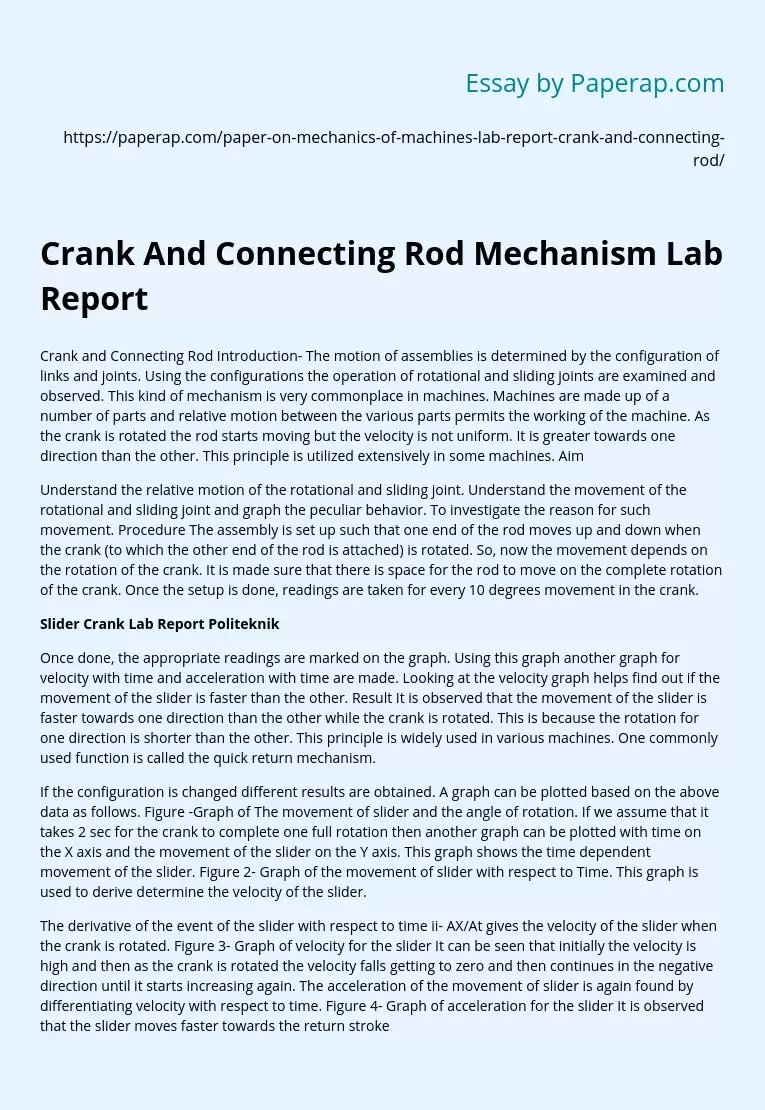# Slider Crank Lab Report Politeknik

Crank and Connecting Rod Introduction- The motion of assemblies is determined by the configuration of links and joints. Using the configurations the operation of rotational and sliding joints are examined and observed. This kind of mechanism is very commonplace in machines. Machines are made up of a number of parts and relative motion between the various parts permits the working of the machine. As the crank is rotated the rod starts moving but the velocity is not uniform. It is greater towards one direction than the other.

This principle is utilized extensively in some machines. Aim

Understand the relative motion of the rotational and sliding joint. Understand the movement of the rotational and sliding joint and graph the peculiar behavior. To investigate the reason for such movement. Procedure The assembly is set up such that one end of the rod moves up and down when the crank (to which the other end of the rod is attached) is rotated. So, now the movement depends on the rotation of the crank.

It is made sure that there is space for the rod to move on the complete rotation of the crank. Once the setup is done, readings are taken for every 10 degrees movement in the crank.

Once done, the appropriate readings are marked on the graph. Using this graph another graph for velocity with time and acceleration with time are made. Looking at the velocity graph helps find out if the movement of the slider is faster than the other. Result It is observed that the movement of the slider is faster towards one direction than the other while the crank is rotated.

Get quality help nowDoctor JenniferVerified

Proficient in: Mechanical Engineering5 (893)

“ Thank you so much for accepting my assignment the night before it was due. I look forward to working with you moving forward ”+84 relevant experts are online

This is because the rotation for one direction is shorter than the other. This principle is widely used in various machines. One commonly used function is called the quick return mechanism.

If the configuration is changed different results are obtained. A graph can be plotted based on the above data as follows. Figure -Graph of The movement of slider and the angle of rotation. If we assume that it takes 2 sec for the crank to complete one full rotation then another graph can be plotted with time on the X axis and the movement of the slider on the Y axis. This graph shows the time dependent movement of the slider. Figure 2- Graph of the movement of slider with respect to Time. This graph is used to derive determine the velocity of the slider.

The derivative of the event of the slider with respect to time ii- AX/At gives the velocity of the slider when the crank is rotated. Figure 3- Graph of velocity for the slider It can be seen that initially the velocity is high and then as the crank is rotated the velocity falls getting to zero and then continues in the negative direction until it starts increasing again. The acceleration of the movement of slider is again found by differentiating velocity with respect to time. Figure 4- Graph of acceleration for the slider It is observed that the slider moves faster towards the return stroke compared to the forward stroke.

This is observed when the crank is rotated in an anti clockwise manner and the results vary when the rotation is clockwise. Conclusion From the performed experiment it is observed that the slider moves when the crank is rotated. Depending upon the configuration of the mechanism and the rotation of the slider, it shows peculiar movement. In this case the slider moved faster towards the return stroke than the forward stroke. This conclusion is exploited in various machines and is termed as quick return mechanism. This is due to the shorter angle of movement for the slider to move one direction than the other.